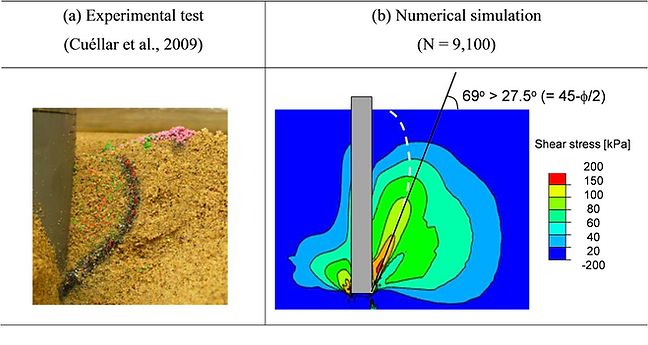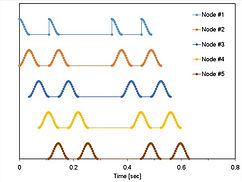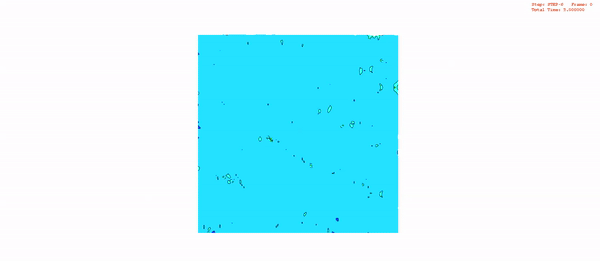Research topics

1. Energy-related Geotechnology

• Numerical study on the energy-related geostructures response

(Offshore foundations and energy piles)

2. Underground Space and Rock Engineering

• Experimental characterization of strain-dependent dynamic properties in a jointed rock mass

• Nonlinear vibration analysis of the resonant column test of granular materials

• Numerical analysis of underground structures considering effective stress and strain nonlinearity

• Numerical study on the evaluation of rail track using a time-variant moving train load

• Development of nondestructive and passive measurement system and evaluation of railway integrity using wireless underground sensor network

4. Others

• Physics-informed models in geotechnical engineering

• Diffusion characteristics of spatially correlated heterogeneous soils

##### a. Experimental characterization of long-term soil response and its application to offshore foundationFigure a1. Monopile foundation response to horizontal repetitive load for different load cycles: (a) Accumulation of horizontal displacement; Distribution of (b) void ratio and (c) deviatoric stress. Note that the deviatoric stress follows the octahedral definition.

Figure a2. Convective granular flow: Comparison between experimentally-observed and numerically-observed shear localization

##### b. Experimental characterization of strain-dependent behavior in a jointed rock mass and its numerical application to underground structuresFigure b1. Equivalent Stress-and Strain-Dependent model in a jointed rock mass

Figure b2. Experimental Setup: Quasi-Static RC

##### c. Numerical study on the evaluation of rail track using a time-variant moving train loadFigure c1. Finite element modeling to implement the moving load applied at the beam nodes. The receiver R is located above the void.Figure c2. Stress fields while a 3000 train class travels along the beam at V=80km/hr.Figure c3. Time, frequency, and time-frequency domain analyses. A train 3000 class load with 4 wheels is applied along the beam node. In the time-frequency domain, the dotted red square in the void condition indicates the reflected stress wave